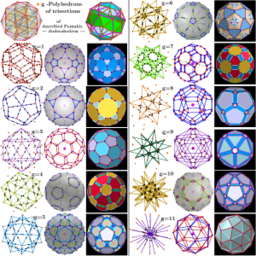# Polyhedron as Polyhedra Generator (segments trisection)

Let the vertices of the initial polyhedron belong to the same sphere. On its basis, can be constructed a certain series of polyhedra. The vertices of each of them are the points of the trisections of the segments of the original polyhedron that have the same length (calculated with a certain accuracy). Obviously, the number of vertices of the constructed polyhedron is twice the number of trisected segments and they all lie on the same sphere.